# RD Sharma Solutions - Chapter 18 - Practical Geometry (Constructions) (Part - 4), Class 8, Maths Notes | Study RD Sharma Solutions for Class 8 Mathematics - Class 8

## Class 8: RD Sharma Solutions - Chapter 18 - Practical Geometry (Constructions) (Part - 4), Class 8, Maths Notes | Study RD Sharma Solutions for Class 8 Mathematics - Class 8

The document RD Sharma Solutions - Chapter 18 - Practical Geometry (Constructions) (Part - 4), Class 8, Maths Notes | Study RD Sharma Solutions for Class 8 Mathematics - Class 8 is a part of the Class 8 Course RD Sharma Solutions for Class 8 Mathematics.
All you need of Class 8 at this link: Class 8

PAGE NO 18.6:

Question 1:

Construct a quadrilateral ABCD in which AB  = 3.8 cm, BC  = 3.0 cm, AD  = 2.3 cm, AC  = 4.5 cm and BD  = 3.8 cm.

Steps of construction:

Step I: Draw AC = 6 cm.

Step II: With A as the centre and radius 3.8 cm, draw an arc.

Step III : With C as the centre and radius 3.0 cm, draw an arc to intersect the arc drawn in Step II at B.

Step IV: With B as the centre and radius 3.8 cm, draw an arc on the other side of AC.

Step V :With A as the centre and radius 2.3 cm, draw an arc to intersect the arc drawn in Step IV at D.

Step VI: Join BA, DA, BC and CD to obtained the required quadrilateral.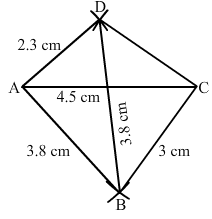Question 2:

Construct a quadrilateral ABCD in which BC  = 7.5 cm, AC  =  AD  = 6 cm, CD  = 5 cm and BD  = 10 cm.

Steps of construction:

Step I: Draw AC = 6 cm.

Step II: With A as the centre and radius 6 cm, draw an arc.

Step III : With C as the centre and radius 5 cm, draw an arc to intersect the arc drawn in Step II at D.

Step IV: With D as the centre and radius 10 cm, draw an arc on the other side of the line segment AC.

Step V :With C as the centre and radius 7.5 cm, draw an arc to intersect the arc drawn in Step IV at B.

Step VI: Join BA, DA, BC and CD to obtained  the required quadrilateral.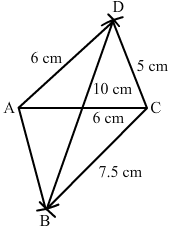Question 3:

Construct a quadrilateral ABCD, when AB  = 3 cm, CD  = 3 cm, DA  = 7.5 cm, AC  = 8 cm and BD  = 4 cm.

If we consider a triangle ABD from the given data, then
AB = 3 cm
BD = 4 cm
AB + BD = 3 + 4 = 7 cm
However, we know that the sum of the lengths of two sides of a triangle is always greater than the third side.
Therefore, construction is not possible from the given data.

Question 4:

Construct a quadrilateral ABCD given AD  = 3.5 cm, BC  = 2.5 cm, CD  = 4.1 cm, AC  = 7.3 cm and BD  = 3.2 cm.

Steps of construction:

Step I: Draw CD = 4.1 cm.

Step II: With C as the centre and radius 7.3 cm, draw an arc.

Step III : With D as the centre and radius 3.5 cm, draw an arc to intersect the arc drawn in Step II at A.

Step IV: With D as the centre and radius 3.2 cm, draw an arc on the other side of AC.

Step V :With C as the centre and radius 2.5 cm, draw an arc to intersect the arc drawn in Step IV at B.

Step VI: Join BA, DA, BC and BD and AC to obtained the required quadrilateral.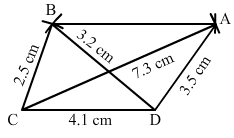Question 5:

Construct a quadrilateral ABCD given AD  = 5 cm, AB  = 5.5 cm, BC  = 2.5 cm, AC  = 7.1 cm and BD  = 8 cm.

Steps of construction:

Step I: Draw AB = 5.5 cm.

Step II: With A as the centre and radius 7.1 cm, draw an arc.

Step III : With B as the centre and radius 2.5 cm, draw an arc to intersect the arc drawn in Step II at C.

Step IV: With B as the centre and radius 8 cm, draw an arc.

Step V :With A as the centre and radius 5 cm, draw an arc to intersect the arc drawn in Step IV at D.

Step VI: Join DA, DB, BC, AC and CD to obtained  the required quadrilateral.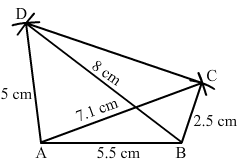Question 6:

Construct a quadrilateral ABCD in which BC  = 4 cm, CA  = 5.6 cm, AD  = 4.5 cm, CD  = 5 cm and BD  = 6.5 cm.

Steps of construction:

Step I: Draw BC = 4 cm.

Step II: With B as the centre and radius 6.5 cm, draw an arc.

Step III : With C as the centre and radius 5 cm, draw an arc to intersect the arc drawn in Step II at D.

Step IV: With C as the centre and radius 5.6 cm, draw an arc on the same side.

Step V :With D as the centre and radius 4.5 cm, draw an arc to intersect the arc drawn in Step IV at A.

Step VI: Join BA, AC, DA, BD and CD to obtained the required quadrilateral.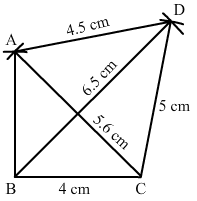The document RD Sharma Solutions - Chapter 18 - Practical Geometry (Constructions) (Part - 4), Class 8, Maths Notes | Study RD Sharma Solutions for Class 8 Mathematics - Class 8 is a part of the Class 8 Course RD Sharma Solutions for Class 8 Mathematics.
All you need of Class 8 at this link: Class 8Use Code STAYHOME200 and get INR 200 additional OFF

## RD Sharma Solutions for Class 8 Mathematics

88 docs

Track your progress, build streaks, highlight & save important lessons and more!

,

,

,

,

,

,

,

,

,

,

,

,

,

,

,

,

,

,

,

,

,

,

,

,

,

,

,

;